## MATHS :: Lecture 20 :: Second order differential equations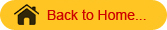Second order differential equations with constant coefficients
The general form of linear Second order differential equations with constant coefficients is(aD2 + bD + c ) y = X                                                (i)
Where a,b,c are constants and X is a function of x.and D =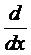When X is equal to zero, then the equation is said to be homogeneous.
Let  D = m Then equation (i) becomes
am2 +bm +c = 0
This is known as auxiliary equation. This quadratic equation has two roots say m1 and m2.
The solution consists of one part namely complementary function
(ie) y = complementary function

Second order DEs1

Second order DEs2

Second order DEs3

Complementary Function
Case (i)
If the roots (m1 & m2) are real and distinct ,then the solution is given by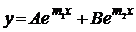where A and B are the two arbitrary constants.
Case (ii)
If the roots are equal say m1 = m2 = m, then the solution is given by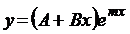where A and B are the two arbitrary constants.
Case (iii)
If the roots are imaginary  say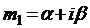and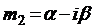Where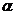and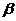are real. The solution is given by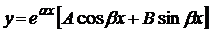where A and B are arbitrary constants.
Particular integral
The equation (aD2 + bD + c )y = X   is called a non homogeneous second order linear equation with constant coefficients. Its solution consists of two terms complementary function and particular Integral.
(ie) y = complementary function + particular Integral
Let the given equation is f(D) y(x) = X
y(x) =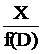Case (i)
Let X=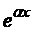and f()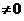Then P.I =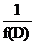=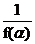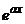Case (ii)
Let X = P(x)   where P(x) is a polynomial
Then P.I =P(x)   =  [f(D)]-1 P(x)
Write [f(D)]-1 in the form (1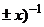(1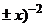and proceed to find higher order derivatives depending on the degree of the polynomial.

Newton's Law of Cooling

Rate of change in the temperature of an object is proportional to the difference between the temperature of the object and the temperature of an environment. This is known as Newton's law of cooling. Thus, if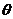is the temperature of the object at time t, then we have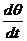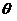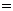-k()
This is a first order linear differential equation.
Population Growth
The differential equation describing exponential growth is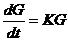This equation is called the law of growth, and the quantity K in this equation is sometimes known as the Malthusian parameter.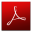Download this lecture as PDF here